# I.E. Irodov Solutions on Molecules and Crystals

Molecules and crystals have a major role in our daily lives. We come across many molecules and crystals in our day to day life. This also has a greater importance in Class 12 Physics. The various molecules, their properties are learnt in this chapter. IE IRODOV Physics Solutions provide solutions to various problems and questions in this chapter. The various kinds of questions mentioned help the students to think at a higher level and build confidence. The numericals are well explanatory with formulae and diagrams.

One can easily get practical knowledge if these sort of numerical problems are solved and understood. The various kinds of molecules, crystal and its structure can be studied by solving several kinds of problems. This type of study enables the students to build interest in studies and enhance their theoretical knowledge. Better understanding, perfect equations and answers are the key points to score higher marks in the upcoming board and competitive examinations.

### I.E. Irodov Solutions on Molecules and Crystals

1. Determine the angular rotation velocity of an S2 molecule promoted to the first excited rotational level if the distance between its nuclei is d = 189 pm.

Solution:

1. In the first excited rotational level J = 1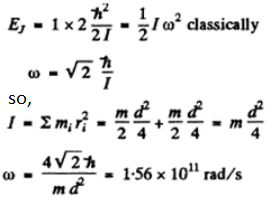where m is the mass of the mole cub and ri is the distance of the atom from the axis.

2. For an HCl molecule find the rotational quantum numbers of two neighbouring levels whose energies differ by 7.86 meV. The nuclei of the molecule are separated by the distance of 127.5 pm.

Solution:

1. The axis of rotation passes through the die centre of mass of the HCl molecule. The distances of the two atoms from the centre of mass are

$d_{H}=\frac{m_{Cl}}{m_{HCl}}d , d_{Cl}=\frac{m_{H}}{m_{HCl}}d$

Thus I - moment of inertia about the axis

$\frac{4}{2}m_{H}d^{2}_{H}+m_{Cl}d^{2}_{Cl}=\frac{m_{H}m_{Cl}}{m_{H}+m_{Cl}}d^{2}$

The energy difference between two neighbouring levels whose quantum numbers are J & J - 1 is $\frac{\not{h}}{2I} 2J=\frac{J\not{h}}{I}=7.86 meV$

Hence J = 3 and the levels have quantum numbers 2 & 3

3. Find the angular momentum of an oxygen molecule whose rotational energy is E = 2.16 meV and the distance between the nuclei is d = 121 pm?

Solution:

1. The angular momentum is

$\sqrt{2IE}=M$

Now

$I=\frac{md^{2}}{4}$

m = mass of O2 molecule = 1.9584 x 10-39 gm c,2

So M=3.68 x10-27erg sec=3.49${\not{h}}$

This corresponds to J = 3

4. Show that the frequency intervals between the neighbouring spectral lines of a true rotational spectrum of a diatomic molecule are equal. Find the moment of inertia and the distance between the nuclei of a CH molecule if the intervals between the neighbouring lines of the true rotational spectrum of these molecules are equal to Δω = 5.47.1012 s-1

Solution:

1. From

$E_{J}=\frac{\not{h}}{2I}J(J+1)$

and the selection rule ΔJ = 1 or J → J - 1 for a pure rotational spectrum we get

$\omega (J, J-1)=\frac{\not{h}J}{I}$

Thus transition lines are equispaced in frequency $\Delta \omega=\frac{\not{h}}{I}$

In the case of CH molecule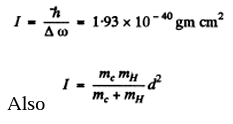Hence, d = 1.117 x 10-8 cm = 111.7 pm

5. For an HF molecule find the number of rotational levels located between the zeroth and first excited vibrational levels assuming rotational states to be independent of vibrational ones. The natural vibration frequency of this molecule is equal to 7.79.1014 rad/s, and the distance between the nuclei is 91.7 pm.

Solution:

1. If the vibrational frequency is to the excitation energy of the first vibrational level will be hωo. Thus if there are J rotational levels contained in the band between the ground state and the first vibrational excitation, then

${\not{h}} \omega_{0}=\frac{J(J+1)\not{h}}{2I}$

whereas stated in the problem we have ignored any coupling between the two. For HF molecule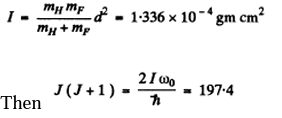For J = 14 , J (J + 1 ) = 210 . For J - 13, J (J + 1) = 182. Thus there lie 13 levels between the ground state and the first vibrational excitation.

6. Evaluate how many lines there are in a true rotational spectrum of CO molecules whose natural vibration frequency is ω = 4.09.1014 s-1 and moment of inertia I = 1.44.10-39g•cm2.

Solution:

1. Calculating $\frac{2I\omega _{o}}{\not{h}}$

We get

$\frac{2I\omega _{o}}{\not{h}}\approx 1118$

Now this must equal $J(J+1)=(J+\frac{1}{2})^{2}$

Taking the square root we get J = 33

7. Find the number of rotational levels per unit energy interval, dN/dE, for a diatomic molecule as a function of rotational energy E. Calculate that magnitude for an iodine molecule in the state with rotational quantum number J = 10. The distance between the nuclei of that molecule is equal to 267 pm.

Solution:

1. From the formula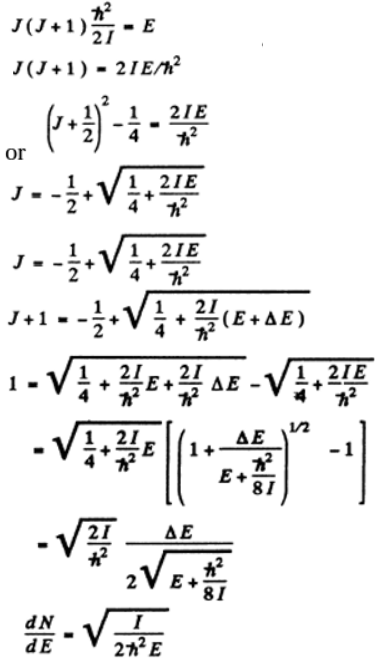*The quantity dN/dE is 1/∆E.

Again,

For an iodine molecule

I = mId2/ 2 = 7*57 x 10-38 gm cm2

Thus for J = 10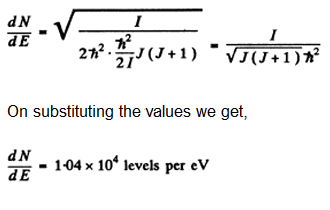8. Find the ratio of energies required to excite a diatomic molecule to the first vibrational and to the first rotational level. Calculate that ratio for the following molecules:

 Molecule W, 1014s-1 d,pm H2 8.3 74 HI 4.35 160 (c) I2 040 267

Here ω is the natural vibration frequency of a molecule, d is the distance between nuclei.

Solution:

1. For the first rotational level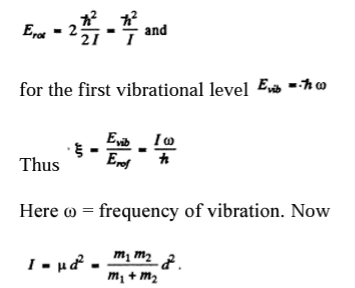(a) For H2 molecule I = 4-58 x 10-41 gm cm2 and $\xi =36$

(b) For HI molecule, I = 4.2 47 x 10-40gmcm2 and $\xi =175$

(c) For I2 molecule I = 7.57 x 10-38gmcm2 and $\xi =2872$

9. The natural vibration frequency of a hydrogen molecule is equal to 8.25.1014 s-1, the distance between the nuclei is 74 pm, Find the ratio of the number of these molecules at the first excited vibrational level (v = 1) to the number of molecules at the first excited rotational level (J = 1) at a temperature T = 875 K. It should be remembered that the degeneracy of rotational levels is equal to 2J + 1.

Solution:

1. The energy of the molecule in the first rotational level will be $\frac{\not{h}}{I}$.The ratio of tire number of molecules at first excited vibrational level to the number of molecules at the first excited rotational level is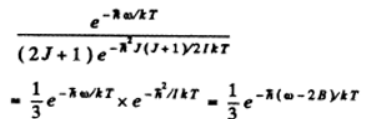We know B = h/2I

fro hydrogen molecule, I = (1/2) mHd2

=4.58 x 10-41 gm cm2

on substituting the values, we get

3.04 x 10-4

10. Derive Equation, making use of the Boltzmann, distribution. From Eq. (6.4c) obtain the expression for molar vibration heat capacity Cv vib of diatomic gas. Calculate Cvvib for Cl2 gas at the temperature of 300 K. The natural vibration frequency of these molecules is equal to 1.064 • 1014 s- 1

Solution:

1. By definition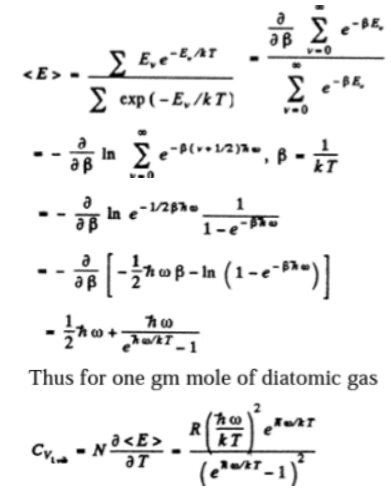where R = Nk is the gas constant

In present case, $\frac{\not{h}w}{KT}=2.7088$

$C_{v_{1}}= 0.56 R$

11. In the middle of the rotation-vibration band of emission spectrum of HCl molecule, where the "zeroth" line is forbidden by the selection rules, the interval between neighbouring lines is Δω = 0.79.10 13 s-1. Calculate the distance between nuclei of the HCl molecule.

Solution:

1. In the rotation vibration band, the main transition is due to a change in vibrational quantum number v → v-1. Together with this rotational quantum number may change. The “Growth line” 0 → 0 is forbidden in this case so the neighbouring lines arise due to 1 →0 or 0 → 1 in the rotational quantum number. Now,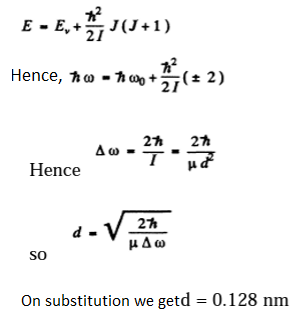12. Calculate the wavelengths of the red and violet satellites, closest to the fixed-line, in the vibration spectrum of Raman scattering by F2 molecules if the incident light wavelength is equal to λ0 = 404.7 nm and the natural vibration frequency of the molecule is ω = 2.15.1014 s-1.

Solution:

1. If λR = wavelength of the red satellite

λv = wavelength of the violet satellite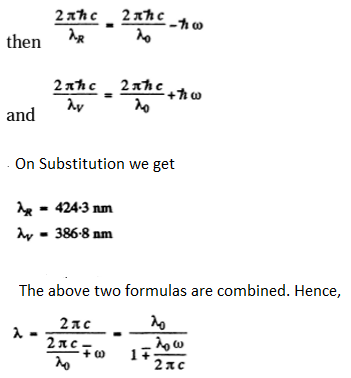13. Find the natural vibration frequency and the quasielastic force coefficient of an S2 molecule if the wavelengths of the red and violet satellites, closest to the fixed-line, in the vibration spectrum of Raman scattering are equal to 346.6 and 330.0 nm.

Solution:

1. As in the previous problem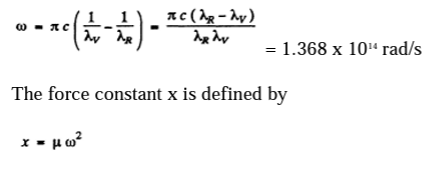where μ= reduced mass of the S2 molecule.

On substitution we get x = 5.01N/cm

14. Find the ratio of intensities of the violet and red satellites, closest to the fixed-line, in the vibration spectrum of Raman scattering by Cl2 molecules at a temperature T = 300 K if the natural vibration frequency of these molecules is ω = 1.06.1014 s-1. By what factor will this ratio change if the temperature is doubled?

Solution:

1. The violet satellite arises from the transition 1→0 in the vibrational state of the scattering molecule while the red satellite arises from the transition 0 → 1. The intensities of these two transitions are in the ratio of initial populations of the two states i.e. in the ratio e-πω/kT.

Thus, Iv/Ir = e-πω/kT. = 0.067

If the temperature is doubled, the ratio increases to 0.259, an increase of 3.9 times.

15. Consider the possible vibration modes in the following linear molecules:

(a) CO2 (O — C—O); (b) C2H2 (H—C —C—H).

Solution:

1. (a) CO2 (O - C - O)

The molecule has 9 degrees of freedom 3 for each atom. This means that it can have up to nine frequencies. 3 degrees of freedom correspond to rigid translation, the frequency associated with this is zero as the potential energy of the system can not change under rigid translation. The P.E. will not change under rotations about axes passing through the C-atom and perpendicular to the O - C - O line. Thus there can be at most four non zero frequencies. We must look for modes different from the above.

The different modes are given below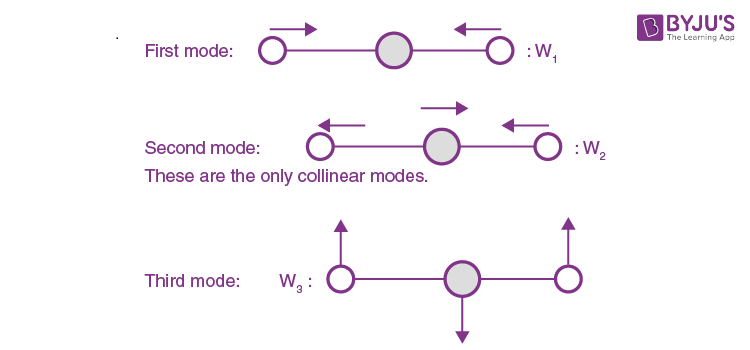(Vibration is in and perpendicular to the plane of paper)

(b) C2H2 ( H - C - C - H )

There are 4 x 3 - 3 - 2 = 7 different vibrations.

There are three collinear modes.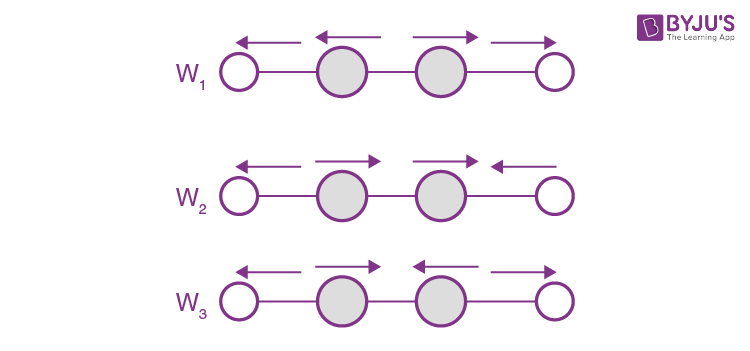Two other doubly degenerate frequencies are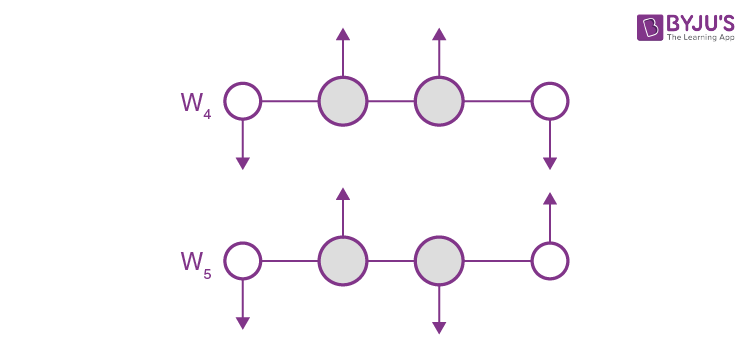together with their counterparts in the plane perpendicular to the plane of paper.

16. Find the number of natural transverse vibrations of a string of length l in the frequency interval from ω to ω + dω if the propagation velocity of vibrations is equal to v. All vibrations are supposed to occur in one plane.

Solution:

1. Suppose the string is stretched along the x-axis from x = 0 to x = l with the endpoints fixed. Suppose y(x, t) is the transverse displacement of the element at x at time t. Then y (x, t) obeys

$\frac{\partial^2 y}{\partial t^2}=v^2\frac{\partial^2 y}{\partial x^2}$

We look for a stationary wave solution of this equation

y(x,t) = A sin(ω/v) x sin(ωt+δ)

where A and δ are constants. In this from y = 0 at x = 0. The further condition y = 0 at x = l.

This implies ωl/v = Nπ, N>0

or N = (l/πv)ω

N is the number of modes of frequency ≤ ω.

Thus, dN = (l/πv)dω

17. There is a square membrane of area S. Find the number of natural vibrations perpendicular to its plane in the frequency interval from ω to ω + dω if the propagation velocity of vibrations is equal to v.

Solution:

1. Let be the displacement of the element at (x, y) at time t. Then it obeys the equation

$\frac{\partial^2 \xi}{\partial t^2}=v^2(\frac{\partial^2 \xi}{\partial x^2} + \frac{\partial^2 \xi}{\partial y^2})$

where ξ = 0 at x = 0, x = l, y = 0 and y = l.

We look for a solution in the form

ξ = A sin k1 x sin k2 y sin(ωt +δ)

Then, ω2 = v2(k12 + k22)

k1 = nπ/l and k2 = mπ/l

Or n2 + m2 = (lω/πv)2

Here n, m > 0 . Each pair (n, m) determines a mode. The total number of modes whose frequency is ≤ ω is the area of the quadrant of a circle of radius $\frac{lw}{\pi v}$ i.e.

N = (π/4) (lω/πv)2

Then, dN = l2/(2πv2) ω dω = [S/(2πv2)] ωdω

where S = I2 is the area of the membrane

18. Find the number of natural transverse vibrations of a right-angled parallelepiped of volume V in the frequency interval from ω to ω + dω if the propagation velocity of vibrations is equal to v.

Solution:

1. For transverse vibrations of a 3-dimensional continuum (in the form of a cube say) we have the equation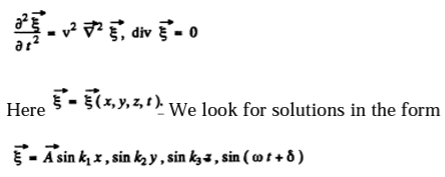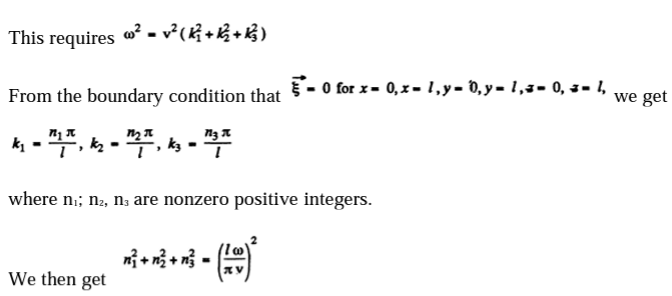Each triplet (n1; n2, n3) determines a possible mode and the number of such modes whose frequency ≤ω is the volume of the all-positive octant of a sphere of radius $\frac{lw}{\pi v}$. Considering also the fact that the subsidiary condition div $\xi =0$ implies two independent values of $\vec{A}=$ for each choice of the wave vector (k1, k2, k3) we find

N(ω) = (1/8) (4π/3) (lω/πv)3 . 2 = Vω3/3π2v3

Thus, dN = [Vω32v3] dω

19. Assuming the propagation velocities of longitudinal and transverse vibrations to be the same and equal to v, find the Debye temperature

(a) for a unidimensional crystal, i.e. a chain of identical atoms, incorporating no atoms per unit length;

(b) for a two-dimensional crystal, i.e. a plane square grid consisting of identical atoms, containing number of atoms per unit area;

(c) for a simple cubic lattice consisting of identical atoms, containing a number of atoms per unit volume.

Solution:

1. To determine the Debye temperature we cut off the high-frequency modes in such a way as to get the total number of modes correctly.

In a linear crystal with no l atoms, the number of modes of transverse vibrations in any given plane cannot exceed noI. Then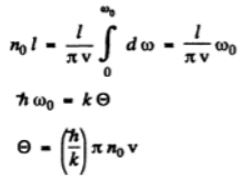(Cut off frequency (ωo) is related to the Debye temperature.)

(b) In a square lattice, the number of modes of transverse oscillations cannot exceed noS. Thus,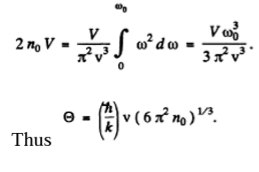20: Calculate the zero-point energy per one gram of copper whose Debye temperature is Θ = 330 K.

Solution:

1. Molar zero point energy is (9/8) R Θ. The zero point energy per gm of copper is (9RΘ/8MCM), MCM is atomic weight of the copper.